### Products

#### Perpenso Calc

A fully paid app.
Five calculators in one: scientific, statistics, business, hex and bill/tip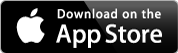#### Perpenso Calc 4

Five calculators in one: scientific, statistics, business, hex and bill/tipYou don't always have a calculator with you butPerpenso® Calc 4 is a calculator application for the Apple iPhone, iPad and iPod touch. It offers five calculators in one upgradable lite app: scientific, statistics, business, hex and bill/tip. Scientific mode is included and the other modes are optional.

Perpenso Calc is a related fully paid app. All modes are included. Perpenso Calc is offered at a discounted price and may cost less than adding all your desired functionality to Perpenso Calc 4.

Both versions are feature rich apps that are comparable to traditional handheld scientific, business and hex calculators.

iOS 4.3 or later is required.

Perpenso Calc 4 includes: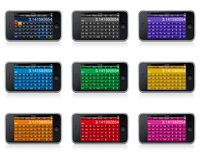• Large readable buttons.
• A calculator tape (except in lite mode).
• A built-in manual.
• Nine color schemes (except in lite mode).

In all calculators Perpenso Calc 4 offers:

• Copy and paste.
• Optional RPN entry (except in lite mode).
• Decimal based arithmetic (no binary rounding).
• 20 digit precision (enough for 64-bit arithmetic).
• 10 memory registers.
• Customizable display lines (iPad only, not available in lite mode)

Scientific mode provides:

• 72 operations (43 complex number).
• Fractions.
• Complex numbers, cartesian (rectangular) and polar.
• 2D rotation.
• Scientific and engineering notation.
• Unit and time conversions.

Statistics mode (an optional In-App Purchase) includes:

• 42 operations.
• Optional worksheet for data entry/editing and viewing common stats.
• Importing data from the web.
• Single and two variable data.
• Descriptive Statistics.
• Linear regression.
• Probabilities.

Business mode (an optional In-App Purchase) offers:

• 53 operations.
• Optional worksheet for data entry/editing and viewing common calculations.
• Breakeven point.
• Profit margin.
• Time value of money.
• Amortization.
• Cash flows.
• Interest rate conversion.
• Running total.

Hex mode (an optional In-App Purchase) provides:

• 43 operations.
• Optional worksheets for data entry/editing and performing common conversions.
• Decimal, hex, octal and binary.
• 64-bit and 32-bit modes.
• Unsigned and signed modes.
• Bitwise, shift and rotate operators.
• Bit set and clear.
• Byte swapping.
• UTF-8, UTF-16 and UTF-32 encoding, decoding and conversions.
• IEEE single and double precision floating point encoding and decoding.
• RGBA decoding with a preview of the color.

In bill / tip mode (an optional In-App Purchase) Perpenso Calc 4 easily determines the portion of a bill that you are responsible for and an appropriate tip. It allows you to:

• Add items to a scrollable list.
• Set item quantity.
• Set the percentage of an item that you are responsible for.
• Set tax and tip percentage.
• Set tax and tip amount.
• Split the total.
• Send itemized bill to calculator tape.
• Enter/edit items and view some calculations on an optional worksheet

# All Modes

Customizable display lines.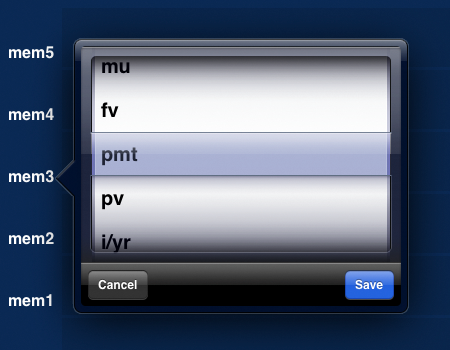On an iPad there are seven user definable display lines. These lines may show additional values on the stack, memory registers or special purpose registers for business and hex modes. Labels appear to the left of the numbers to identify the value currently displayed. Tapping on the label will allow you to change the value. Or use buttons that will redefine all the user definable display lines according to a particular theme. Stack, memory, break even point, profit margin, time value of money and hex, octal, binary and unicode conversions.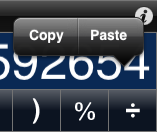Works with other apps.

Copy and paste are convenient ways to transfer data to and from other applications such as Notes and Mail. The numeric value may be a decimal number, a fraction or a complex number in cartesian or polar form.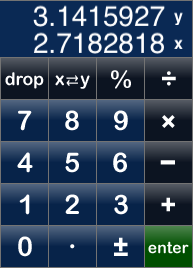Efficient and explicit calculations.

Reverse Polish Notation (RPN), also known as postfix notation, is an optional entry method that gives you explicit control over calculations without using parenthesis. RPN is a favorite of many handheld calculator users. Not available in lite mode.

20 digit precision, enough for 64-bit arithmetic.Can your calculator calculate 264 (2 yx 64) = 18446744073709551616? Very few can, they lose lower end digits and display a truncated value using exponential notation. Many calculator apps use the hardware floating point unit (FPU). While this is perfectly fine for many applications and games it is, in our opinion, insufficient for a calculator. The FPU is limited to around 16 decimal digits of precision, far fewer than the 20 needed to perform 64-bit arithmetic. Regardless of how many fractional digits Perpenso Calc 4 is configured to display it is always using 20 digits of precision internally.

Decimal arithmetic, no binary rounding.

Can other calculator apps calculate 0.5 − 0.4 − 0.1 = 0? Surprisingly many cannot and display a very small number rather than zero. This is due to another problem with the FPU, it performs binary arithmetic rather than the decimal arithmetic that people use. As numbers are converted between binary and decimal small rounding errors can occur. Handheld calculators use decimal arithmetic, not binary, to avoid such rounding errors. So does Perpenso Calc 4.

# Scientific Mode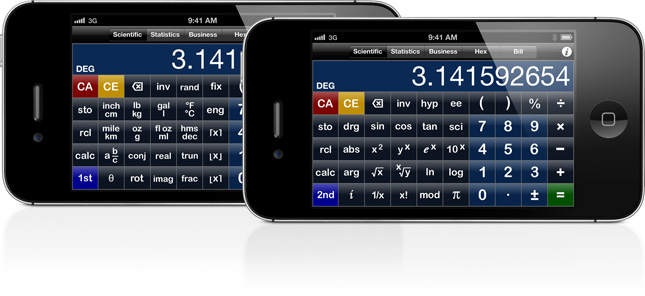72 operations, 43 of the calculations support complex numbers (marked with c).

• Addition c, subtraction c, multiplication c and division c.
• Reciprocal c.
• Modulo.
• Percent c.
• Negate c.
• Sign c.
• Truncate c, fractional component c, absolute value c, round c, floor c and ceiling c.
• Square c, square root c, power c and xth root c.
• Factorial.
• Natural exponential c, natural logarithm c, common exponential c and common logarithm c.
• Sine c, cosine c and tangent c. Hyperbolic c and inverse c.
• Real part c and imaginary part c.
• Magnitude c and argument (angle) c.
• Conjugate c.
• Rotate c.
• Cartesian (rectangular) and polar conversions c.
• Random number.

• Decimal / fraction conversions.
• Inch / cm conversions.
• Mile / kilometer conversions.
• Pound / kilogram conversions.
• Ounce / gram conversions.
• Gallon / liter conversions, US and Imperial.
• Fluid ounce / milliliter conversions, US and Imperial.
• Fahrenheit / Celsius conversions.
• Hours (or degrees), minutes and seconds / decimal conversions.

• Store and recall.
• Last result and last x.
• Drop X, swap XY, roll up and roll down.

Seamless support for fractions.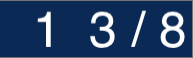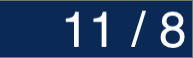In Perpenso Calc 4 fractions are generally interchangeable with decimal numbers. Nearly all calculations accept fractions and will return a fraction when the operands are fractions. Fractions may be displayed as, and converted between, proper and improper forms.

Better support for complex numbers than many handheld calculators.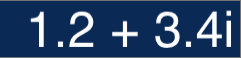Most mathematical operations in Perpenso Calc 4 accept complex numbers, more operations than many handheld calculators. Complex numbers may be displayed as, and converted between, cartesian (rectangular) and polar forms. Points in the complex plane may be rotated about the origin.

# Statistics Mode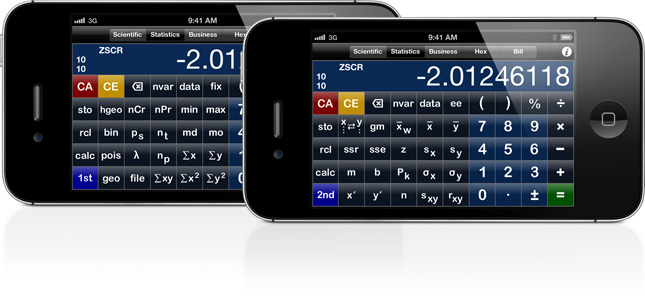42 operations.

• Optional worksheet for data entry/editing and viewing common statistics.
• Importing data from the web.
• Single and two variable data modes.
• Swap XY data.

• Mean, weighted mean and geometric mean.
• Z-score.
• Median and mode.
• Min and max.
• Standard deviations.
• Covariance and correlation.
• Percentile.
• Sums.

• Slope.
• Y-intercept.
• Predicted x/y.
• SSE.
• SSR.

• Combinations.
• Permutations.
• Geometric, hypergeometric, poisson and binomial probability functions.

A statistics worksheet for more natural editing.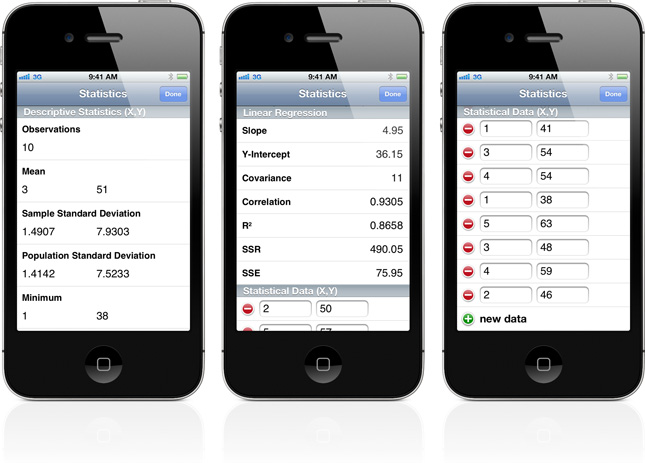While the button based interface of a traditional handheld calculator works well for many things it is awkward for some tasks. Editing data is one such task. Fortunately iOS provides a modern and powerful graphical interface.

Worksheets offer a more natural way to enter, edit and view data and to perform some common calculations. In the statistics worksheet data may be entered and edited in a table. Above this table descriptive statistics such as mean and standard deviation are automatically displayed. In two variable mode a linear regression is also automatically displayed.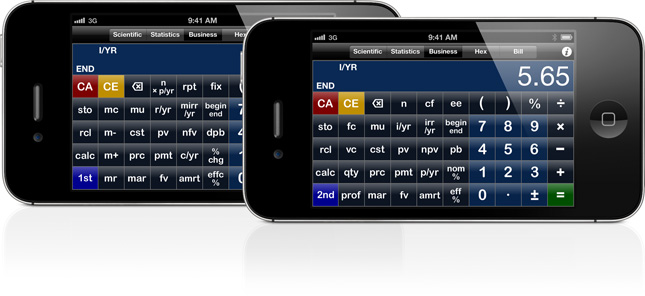53 operations.

• Optional worksheet for data entry/editing and viewing common calculations.
• Breakeven point.
• Profit margin.
• Time value of money.
• Amortization.
• Cash flows.
• Interest rate conversion.
• Running total.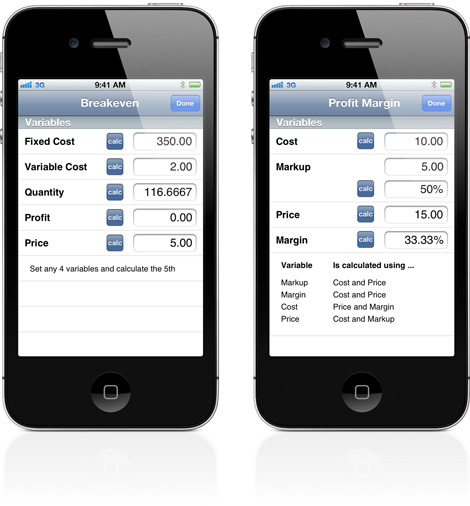In breakeven calculations you can set any four of the five variables and calculate the fifth.

In profit margin calculations a particular pair of variables is set in order to calculate the desired value.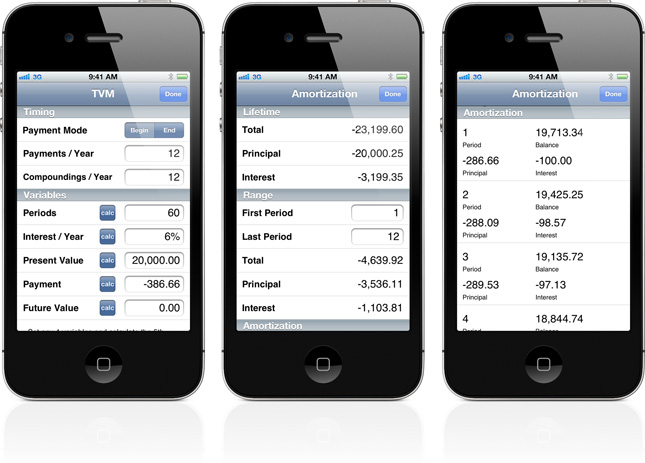Time Value of Money (TVM) calculations can solve a wide range of problems. Loans, mortgages, leases, savings, annuities, etc. There are five key variables. Set any four of these variables and the fifth may be calculated.

Once a TVM calculation has been performed the amortization worksheet may be used to see how the remaining balance, principal payments and interest payments change over time. You can also see the amount of interest and principal paid over the life of the loan or during a specific period of time.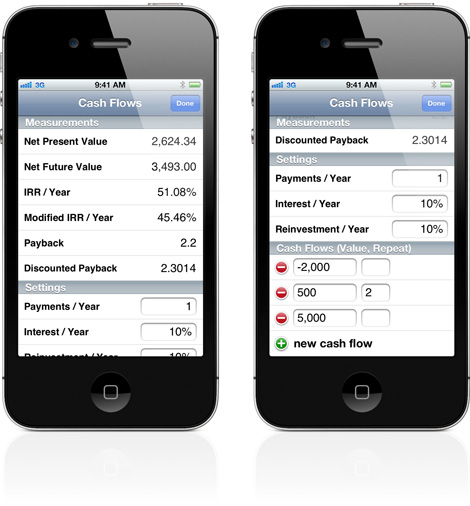In the cash flow worksheet cash flows may be entered and edited in a table. Traditional measurements such as net present value, internal rate of return, payback, etc are automatically calculated and displayed.

# Hex Mode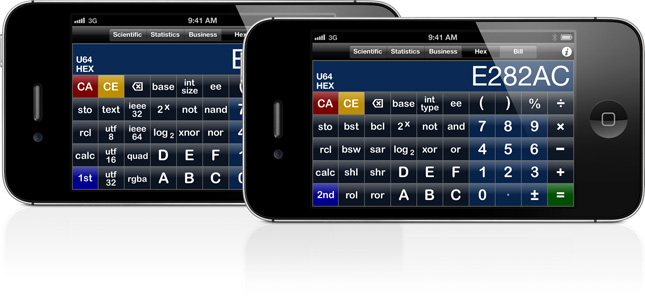43 operations.

• Optional worksheets for data entry/editing and performing common conversions.
• Decimal, hexadecimal, octal and binary conversions.
• 32-bit and 64-bit conversions.
• Unsigned and signed conversions.

• Binary exponential and binary logarithm.
• Not, and, or, exclusive or, nand, nor, xnor.
• Bit set and clear.
• Byte swap.
• Logical shift left and right.
• Arithmetic shift right.
• Rotate left and right.

• Text to UTF-8.
• UTF-8, UTF-16 and UTF-32 to text.
• Value to IEEE 32 or IEEE 64.
• IEEE 32 or IEEE 64 to value.
• Value to RGBA and color.Work with various Unicode character encodings.

Perpenso Calc 4 allows you to convert to and from 8-, 16- and 32-bit Unicode character encodings. Enter a character at the keyboard and find its UTF-8, UTF-16 or UTF-32 encoding. Or enter a numeric value representing a character encoding and find out what character it is. You may also convert from one encoding to another, for example UTF-16 to UTF-8.

Decode and visualize RGB values with alpha.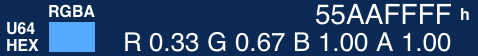Enter numeric values representing RGBA colors and find the intensity of the color and alpha channels. There is also a color preview. The background for this preview includes white and black panels so that the alpha effect may be more easily recognized. In the example above the same color is shown at 100% and 50% alpha.

Encode and decode IEEE floating point values.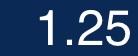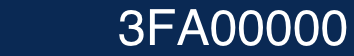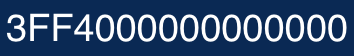IEEE single precision (32-bit) and double precision (64-bit) floating point values may be converted to and from their hex encodings. You may enter decimal floating point values or hex encodings.Support for dotted quad notation.

You may convert numeric values to dotted quad (dot-decimal) notation. You may also enter numeric values using dotted quad notation.

A hex worksheet for simple conversions.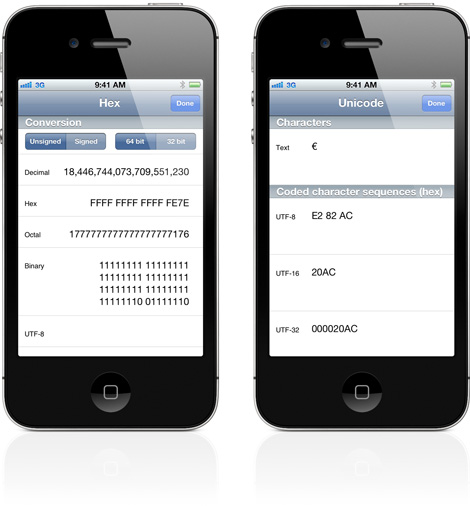The hex worksheet provides various fields showing how a value may be represented. You may also reinterpret values as signed or unsigned or as 64-bit or 32-bit.

In the unicode worksheet you may enter characters in the text field and the various UTF encodings will be automatically displayed. Hex values may be edited and the other fields are automatically updated.

# Bill / Tip Mode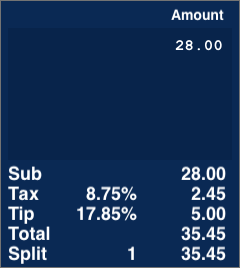Quickly determine a tip.

Perpenso Calc 4 allows you to specify the details of your bill but does not require you to do so. Want to quickly determine a tip? Simply enter your total as an item and a tip will be calculated using your default tip rate.

If you would like to change the tip you may enter a tip percentage or if you prefer you may specify the tip amount.Determine the portion of a bill that you are responsible for and a tip.

Optionally specifying the percentage of an individual item that you are responsible for provides functionality that simply splitting the total does not. You may calculate the actual portion of a bill that you are responsible for. For example if you shared an appetizer with three friends, had an entree and two drinks simply enter the full price of the appetizer and your percentage of 25, the price of your entree and then the price of your drink and a quantity of 2.

Convenient editing using a bill worksheet.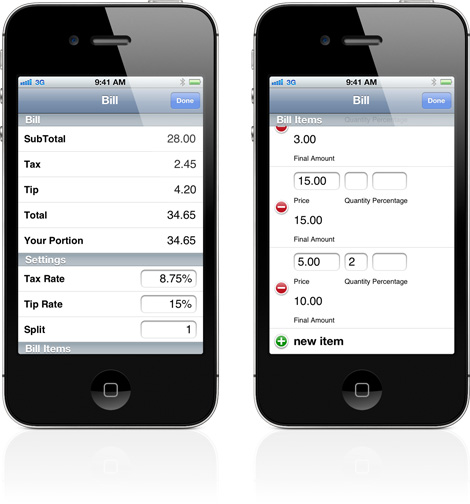The bill worksheet provides a convenient way to enter, edit and view the various bill amounts. At the top of the worksheet the calculated amounts are automatically displayed. Below are editable fields for the tax rate, tip rate, spit and item amounts.

# Tape, Manual and Settings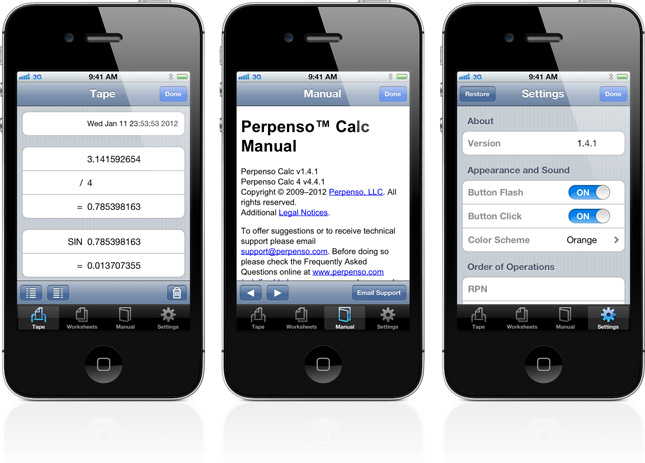On its flip side Perpenso Calc 4 offers a calculator tape (except in lite mode), worksheets, a built-in manual and settings controls. The tape allows you to position operations on the left or right. A double tap allows you to copy the tape. The built-in manual is designed for the iPhone display. A downloadable PDF-based manual with additional content is available.